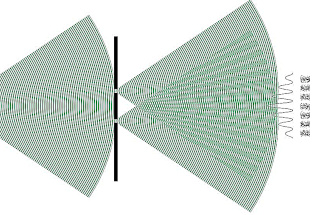Automatic translation Category: matter and particles
Updated March 22, 2022### Image: Interference concerns all quantum particles. Interferences have been observed with electrons, photons, neutrons, atoms and even molecules. These interference phenomena, typical of waves, are all observed experimentally in the microscopic world but disappear in the classical macroscopic world. Two waves of the same type of propagation (mechanical or electromagnetic) are capable of being added together. It is for this reason that we observe interference. As soon as the particle arrives in the classical world, its energy is suddenly reduced to a point on the screen which can then be located because it has taken on the appearance of a corpuscle. It is after the reduction of the wave packet that one sees on the screen the points of impact of the particles sent one by one on the Young slits.

 Energy levels of the electron in the atom H n=1 Fundamental level, lowest energy E1=-13.6/12 =-13.6 eV n=2 First excited level E2=-13.6/22 =-3.4 eV n=3 Second excited level E3=-13.6/32 =-1.51 eV n=4 Third excited level E4=-13.6/42 =-0.85 eV n=5 Fourth excited level E5=-13.6/52 =-0.54 eV n=6 Fifth excited level E6=-13.6/52 =-0.38 eV

### Image: Schrödinger's equation devised in 1925 is a fundamental equation in non-relativistic quantum mechanics. If we solve the equation, we know everything about the system. H(t)|Ψ(t)> = iℏ d/dt |Ψ(t)> allows for example to calculate precisely all the values of the energy that a hydrogen atom can take.

 1997 © Astronoo.com − Astronomy, Astrophysics, Evolution and Earth science. Contact    Mentions légales